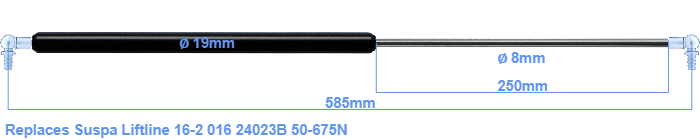# Replacement for Suspa Liftline 16-2 016 24023B 50-675N

\$42.45

Replacement gas spring for the Suspa Liftline 16-2 016 24023B 50-675 Newton. This fits a (possibly already present) ball with a diameter of 10mm. A corresponding ball is included. Brand: Stabilus Industry Line.
 Force Choose an option50 Newton60 Newton80 Newton100 Newton120 Newton140 Newton150 Newton160 Newton180 Newton200 Newton220 Newton240 Newton250 Newton260 Newton280 Newton300 Newton320 Newton340 Newton350 Newton360 Newton380 Newton400 Newton420 Newton440 Newton450 Newton460 Newton480 Newton500 Newton520 Newton540 Newton550 Newton560 Newton580 Newton600 Newton620 Newton640 Newton650 Newton660 Newton675 NewtonClearThe diameter of the cylinder of this gas spring is 19 mm. The cross section of the rod is 8 mm. The stroke is 250 millimeter long. The total length of this gas strut is 585 mm. Keep in mind that this is the length between the centers of the mounting parts. The length from thread to thread (exlcuding the mounting parts) is 545 millimeter. The force of this replacement is 50-675 Newton. This gas spring can replace a Suspa Liftline gas spring, but is not an orignal Suspa Liftline one. HAHN Gasfedern, a Stabilus subsidiary, is responsible for the production of this replacement gas spring. The gas spring has the brand Stabilus Industry Line. Nevertheless, the force and dimensions equal.
Category: